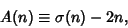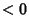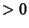## Abundance

The abundance of a numberis the quantitywhereis the Divisor Function. Kravitz has conjectured that no numbers exist whose abundance is an Odd Square (Guy 1994).

The following table lists special classifications given to a numberbased on the value of.NumberDeficient NumberAlmost Perfect Number 0 Perfect Number 1 Quasiperfect NumberAbundant Number